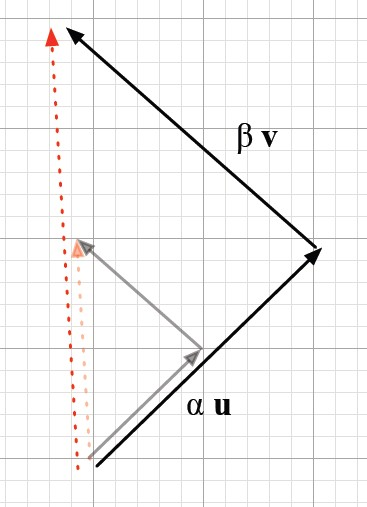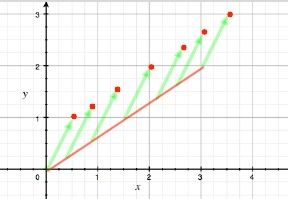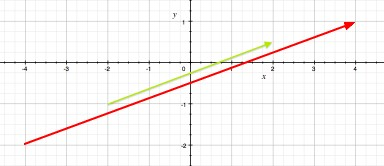# Number System - Real Number (Scalar) -

A real number $\mathbb{R}$ is a value that represents a quantity along a line.

They have been called real to distinguish them from imaginary number.

The adjective real in this context was introduced in the 17th century by René Descartes, who distinguished between real and imaginary roots of polynomials.

The real numbers include all the rational numbers, such as:

• the integer −5
• the fraction 4/3,
• the irrational numbers, such as √2 (1.41421356…)

Real numbers can be thought of as points on an infinitely long line called the number line or real line, where the points corresponding to integers are equally spaced.

Any real number can be determined by a possibly infinite decimal representation, such as that of 8.632.

Since the set of rational numbers is countable, and the set of real numbers is uncountable, almost all real numbers are irrational

## Scalar

In linear algebra, real numbers are called scalar

Greek letters ($\alpha, \beta, \gamma$ ) denote scalars.

## Associativity

real numbers are associative, meaning that for any real numbers x, y, and z, it’s always the case that (x + y) + z = x + (y + z).

## Documentation / Reference

Discover MoreComputer - Floating-point (Representation and usage)

Computer representations of floating point numbers typically use a form of rounding to significant figures, but with binary numbers. The number of correct significant figures is closely related to the...Data Type - Scalar Variable

A scalar variable is an atomic quantity that can hold only one value at a time A scalar function returns a scalar. The contrary of scalar variable are collections. A scalar multiplication is usually...Function - Scalar

Scalar functions return a single value (as opposed to an array). Mathematics definition: Any function whose domain is a vector space and whose value is its scalar field. Is the result of a scalar function...Geometry - Line

A value that represents a quantity along a line is called a real numberLinear Algebra - Orthogonality (Perpendicular)

Orthogonal is linear-algebra-ese for perpendicular. The squared length of the “hypotenuse” (the vector u + v) is Thus following the Pythagorean Theorem u and v are orthogonal when then:...Linear Algebra - (Line|Line Segment)

in linear algebra. Line and line segment definition in Linear Algebra that goes: A line has a dimension of one because only one coordinate is needed to specify a point on it Line_segmentline...Linear Algebra - Linear Equation

A linear equation represents a linear function that forms a straight line. A common form of a linear equation in the two variables (two dimensions) x and y is where: m is a constant named the slope...Linear Algebra - Scalar

Real number in linear algebra are called Scalar_(mathematics)scalars and relates to vectors in a vector space through the operation of scalar multiplication. Greek letters () denote scalars.Linear Algebra - Scalar (Multiplication|Product) - Scaling

Scalar Multiplication (Scaling) is the multiplication of a vector (for instance ) by a scalar (real number) (for instance ) to produce another vector (for instance ) Multiplying a vector v by a scalar...Linear Algebra - Vector Space (set of vector)

A vector space is a subset of the set of function representing a geometric objectpassing through the origin. A vector space over a field F is any set V of vector : with the addition and scalar-multiplication...